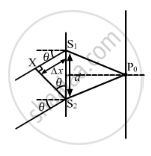Department of Pre-University Education, KarnatakaPUC Karnataka Science Class 12
Advertisement Remove all ads

# A Parallel Beam of Monochromatic Light is Used in a Young'S Double Slit Experiment. the Slits Are Separated by a Distance D and the Screen is Placed Parallel to the Plane of the Slits. - Physics

Sum

A parallel beam of monochromatic light is used in a Young's double slit experiment. The slits are separated by a distance d and the screen is placed parallel to the plane of the slits. Slow that if the incident beam makes an angle $\theta = \sin^{- 1} \left( \frac{\lambda}{2d} \right)$ with the normal to the plane of the slits, there will be a dark fringe at the centre P0 of the pattern.

Advertisement Remove all ads

#### Solution

Let the two slits are S1 and S2 with separation d as shown in figure.The wave fronts reaching P0 from S1 and S2 will have a path difference of S1X = ∆x.

In ∆S1S2X,

$\sin\theta = \frac{S_1 X}{S_1 S_2} = \frac{∆ x}{d}$

$\Rightarrow ∆ x = d\sin\theta$

Using $\theta = \sin^{- 1} \left( \frac{\lambda}{2d} \right)$ we get,

$\Rightarrow ∆ x = d \times \frac{\lambda}{2d} = \frac{\lambda}{2}$

Hence, there will be dark fringe at point P0 as the path difference is an odd multiple of $\frac{\lambda}{2}.$

Is there an error in this question or solution?
Advertisement Remove all ads

#### APPEARS IN

HC Verma Class 11, 12 Concepts of Physics 1
Chapter 17 Light Waves
Q 20 | Page 381
Advertisement Remove all ads

#### Video TutorialsVIEW ALL 

Advertisement Remove all ads
Share
Notifications

View all notifications

Forgot password?